Courses

# Test: Level - 2 Isolation - 1

## 30 Questions MCQ Test Chemistry Class 12 | Test: Level - 2 Isolation - 1

Description
This mock test of Test: Level - 2 Isolation - 1 for Class 12 helps you for every Class 12 entrance exam. This contains 30 Multiple Choice Questions for Class 12 Test: Level - 2 Isolation - 1 (mcq) to study with solutions a complete question bank. The solved questions answers in this Test: Level - 2 Isolation - 1 quiz give you a good mix of easy questions and tough questions. Class 12 students definitely take this Test: Level - 2 Isolation - 1 exercise for a better result in the exam. You can find other Test: Level - 2 Isolation - 1 extra questions, long questions & short questions for Class 12 on EduRev as well by searching above.
QUESTION: 1

### Formation of metallic copper from the sulphide ore in the commercial thermo-metallurgical process essentially involves which one of the following reaction :

Solution:

Self reduction process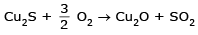2Cu2O + Cu2S → 6Cu + SO2

QUESTION: 2

### Ag2S + NaCN + Zn →Ag This method of extraction of Ag by complex formation and then its displacement is called :

Solution:

The process mention is rxn is known as Mc.
Arthur forest Method :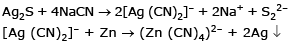QUESTION: 3

### Calcination is the process of heating the ore :

Solution:
QUESTION: 4

Which of the following does not contain Mg :

Solution:
QUESTION: 5

Match the method of concentration of the ore in column I with the ore in column II and select the correct alternate: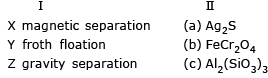Solution:

Magnete seperation → FeCr2O4
froath floatation → Ag2S
gravity separation → Al2 (SiO3)2

QUESTION: 6

Bessemerization is carried out for

(I) Fe
(II) Cu
(III) Al
(IV) silver

Solution:

Bessermerisatioin process is used for Fe and Cu

QUESTION: 7

Refining of silver is done by :

Solution:

By cupellation it is urea for the removal of Pb from Ag and Au

QUESTION: 8

Which of the following is not used for obtaining Ag

Solution:
QUESTION: 9

Solution:

Blister copper is 98 % pure Cu

QUESTION: 10

Which one of the following is not a method of concentration of ore?

Solution:

S melting is not a process of concn of ore.

QUESTION: 11

In which of the following isolations no reducing agent is required :

Solution:

Mercury from cinnarbar (HgS)
(Auto reductant and self reduction) (Cu2S, PbS, HgS)

QUESTION: 12

Chemical leaching is useful in the concentration of :

Solution:

leaching is used for bauxite

QUESTION: 13

The element which could be extracted by electrolytic reduction of its oxide dissolved in a high temperature melt is :

Solution:

Aluminium oxide could be extracted by electrolysis phenomenon.

QUESTION: 14

Consider the following statements :

Roasting is carried out to :

(i) convert sulphide to oxide and sulphate (ii) remove water of hydration

(iii) melt the ore (iv) remove arsenic and sulphur impurities

Of these statements :

Solution:
QUESTION: 15

Iron obtained from blast furance is :

Solution:

Iron obtained from blast furnace is pig iron

QUESTION: 16

Which one of the following statements is not correct :

Solution:

(A) Malachite Cu(OH)2 . CuCO3
(B) Calamine ZnCO3
(C) Cerussite PbCO3
Stellite is an ore

QUESTION: 17

Which of the following is not an ore :

Solution:

Some transition metal can form poly nuclear metal carbonyls also.

QUESTION: 18

In the extraction of nickel by Mond process, the metal is obtained by :

Solution:

Thermal decomposition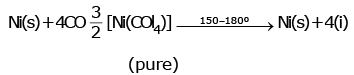QUESTION: 19

B4C(boron carbide) is used except:

Solution:
QUESTION: 20

Froath floatation process is based on:

Solution:
QUESTION: 21

When ZnS and PbS minerals are present together, then NaCN is added to separate them in the froth floatation process as a depressant, because

Solution:

PbS + NaCN/KCN → No. reaction
ZnS + NaCN/KCN → [Zn(CN)4]2– + S2– + 4Na+/ 4K+

QUESTION: 22

When copper is purified by electrorefining process, noble metals like Ag and Au are found in

Solution:

Cu and Ag are found in anode mud.

QUESTION: 23

Formation of Ni(CO)4 and subsequent its decomposition into Ni and CO (recycled) makes basis of Mond's process

Ni + 4CONi (CO)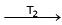Ni + 4CO

T1 and T2 are :

Solution:

Ni + 4CO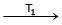Ni (CO)Ni + 4CO
T1 = 50ºC
T2 = 230ºC

QUESTION: 24

Match column–I (process) with column–II (electrolyte) :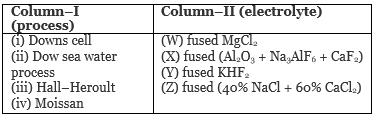Choose the correct alternate.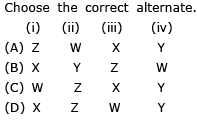Solution:

Down cell → Fused (40 % NaCl + 60 % CaCl2)
Dow seawater process → fused NaCl2
Hall-heroult ⇒ fused (Al2O3 + Na3AlF6 + CaF2)
Moissan → Fused KHF2

QUESTION: 25

(I) FeCr2O4 + NaOH + air → (A) + Fe2O3

(II) (A) + (B) → Na2Cr2O7

(III) Na2Cr2O7 + X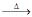Cr2O3

(IV) Cr2O3 + YCr

Q.

Compounds (A) and (B) are :

Solution:

A → Na2CrO4
B → H2SO4

QUESTION: 26

(I) FeCr2O4 + NaOH + air → (A) + Fe2O3

(II) (A) + (B) → Na2Cr2O7

(III) Na2Cr2O7 + XCr2O3

(IV) Cr2O3 + YCr

Q.

(X) and (Y) are :

Solution:

X → CO
Y → Al

QUESTION: 27

(I) FeCr2O4 + NaOH + air → (A) + Fe2O3

(II) (A) + (B) → Na2Cr2O7

(III) Na2Cr2O7 + XCr2O3

(IV) Cr2O3 + YCr

Q.

Na2CrO4 and Fe2O3 are separated by :

Solution:
QUESTION: 28

(I) FeCr2O4 + NaOH + air → (A) + Fe2O3

(II) (A) + (B) → Na2Cr2O7

(III) Na2Cr2O7 + XCr2O3

(IV) Cr2O3 + YCr

Q.

High temperature (> 1000ºC) electrolytic reduction is necessary for isolating :

Solution:
QUESTION: 29

In froth-floatation process, palm oil functions as :

Solution:

Palm oil function as frother. It decreases the surface tension of the total solution, by which the formation of froth becomes easier.

QUESTION: 30

Collector are the substances which help in attachement of an ore particle to air bubble in froth. A popular collector used industrially is :

Solution: